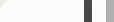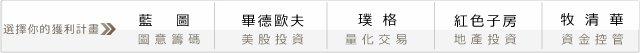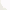## 交易與賭局的當道投資學: Optimal f 實作!

Optimal F的概念是，如果你有一組損益向量，例如銅板賭局的(-1,+2)，這意味著有50%的機會賠光(-1)，有50%的機會賺2倍(+2)，假設未來的損益都是從這個向量去取樣(sample)的話，那你的最佳下注比例為何?

PL=c(-1,2)                                       ##輸入損益向量

if (sum(PL >= 0) == length(PL)) {
OptF = sign(sum(PL))
## 如果全勝(沒賠)，則全壓(f=100%)
}else{

BidF = seq(0, 1, 0.01)                    ##賭0%到100%
TWR = setNames(BidF, BidF)     ##設定TWR向量

for (f in BidF){
TWR[[as.character(f)]] =
(prod(1+f*-PL/min(PL)))^(1/length(PL))
}

##上面部分為計算TWR的關鍵，請參考Vince著作

OptF=as.numeric(names(which.max(TWR)))
##取出Optimal f
}

if (OptF!=1){   ##如果不是全壓，畫下面的圖

plot(TWR, type="l", col="red", lwd=2
, main=paste("Optimal f is", OptF*100, "%"))

abline(h=1, col="green", lwd=2)
##各下注比例底下的TWR

}else{ paste("Optimal f is 100%")
##如果是全壓，show出最佳下注比例為何

http://www.bituzi.com/2015/02/Vince-Optimal-f.html

#### 0 意見: